• 吴恩达机器学习课程->神经网络练习 关于练习的详细内容以及神经网络的原理在这里就不再叙述了。 octave代码下载 神经网络Python实现代码： import scipy.io as sio import numpy as np import ...
练习题背景：网易云课堂->吴恩达机器学习课程->神经网络练习题
关于练习题的详细内容以及神经网络的原理在这里就不再叙述了。
octave代码下载
神经网络Python实现代码：
import scipy.io as sio
import numpy as np
import matplotlib.pyplot as plt
from scipy.optimize import minimize

def displayData(data):
"""
数据可视化
:param data:
:return:
"""
rows = np.int(np.sqrt(data.shape))
cols = np.int(data.shape / rows)

width = np.int(np.sqrt(data.shape))

fig, ax_array = plt.subplots(rows, cols, sharex='all', sharey='all', figsize=(8, 8))

for r in range(rows):
for c in range(cols):
ax_array[r, c].matshow(data[r * rows + c].reshape(width, width), cmap='gray_r')

plt.xticks()
plt.yticks()
plt.show()

def labelMapping(a):
"""
标签映射
:param a:
:return:
"""
zeros = np.zeros(10)
zeros[a - 1] = 1

return zeros

def sigmoid(z):
"""
sigmoid函数
:param z:
:return:
"""

return 1.0 / (1 + np.exp(-z))

"""
sigmoid求导
:param z:
:return:
"""

return sigmoid(z) * (1 - sigmoid(z))

def randInitializeWeights(L_out, L_in):
"""
生成随机权重矩阵
:param L_out:
:param L_in:
:return:
"""
epsilon_init = 0.12
# 服从均匀分布的随机权重矩阵
return np.random.uniform(-epsilon_init, epsilon_init, (L_out, L_in + 1))

def debugInitializeWeights(fan_out, fan_in):
"""
生成调试用的参数矩阵
:param fan_out:
:param fan_in:
:return:
"""
W = np.zeros((fan_in + 1, fan_out))
return np.sin(range(1, W.size + 1)).reshape(W.shape).T / 10

"""
梯度检验
:param lamb:
:return:
"""
input_layer_size = 3
hidden_layer_size = 5
num_labels = 3
m = 5

Theta1 = debugInitializeWeights(hidden_layer_size, input_layer_size)
Theta2 = debugInitializeWeights(num_labels, hidden_layer_size)

X = debugInitializeWeights(m, input_layer_size - 1)

y = 1 + np.mod(range(1, m + 1), num_labels).reshape(m, 1)

y_m = np.zeros((m, num_labels))
for i in range(m):
zeros = np.zeros((num_labels,))
zeros[y[i] - 1] = 1
y_m[i] = zeros

params = np.r_[Theta1.flatten(), Theta2.flatten()]

perturb = np.zeros(params.shape)

e = pow(10, -4)

for p in range(params.size):
perturb[p] = e
loss1 = costFunction(params - perturb, input_layer_size, hidden_layer_size, num_labels, X, y_m, lamb)
loss2 = costFunction(params + perturb, input_layer_size, hidden_layer_size, num_labels, X, y_m, lamb)

numgrad[p] = (loss2 - loss1) / (2 * e)
perturb[p] = 0

print(diff)

def feedForward(Theta1, Theta2, X):
"""
前向传播
:param Theta1:
:param Theta2:
:param X:
:return:
"""
m = X.shape

a1 = np.c_[np.ones(m), X]

# 隐含层, @表示矩阵点乘
z2 = a1 @ Theta1.T
a2 = np.c_[np.ones(m), sigmoid(z2)]

# 输出层
z3 = a2 @ Theta2.T
a3 = sigmoid(z3)

return a1, z2, a2, z3, a3

def costFunction(nn_params, input_layer_size, hidden_layer_size, num_labels, X, y, lamb):
"""
损失函数计算，这里只针对简单的三层神经网络模型
:param nn_params: 展开的所有模型参数
:param input_layer_size: 输入层神经元数量
:param hidden_layer_size: 隐含层神经元数量
:param num_labels: 标签数量，也就是输出层神经元数量
:param X: 样本
:param y: 标签
:param lamb: 规则化系数 lambda
:return: J，损失
"""
# 样本数量
m = X.shape

# 先将展开的所有模型参数，根据神经网络的结构，分解成对应的参数矩阵
Theta1 = nn_params[:hidden_layer_size * (input_layer_size + 1)].reshape((hidden_layer_size, input_layer_size + 1))
Theta2 = nn_params[hidden_layer_size * (input_layer_size + 1):].reshape((num_labels, hidden_layer_size + 1))

_, _, _, _, h = feedForward(Theta1, Theta2, X)

# 计算损失，在矩阵计算中，*表示矩阵的对应元素相乘
J = np.sum((-y) * np.log(h) - (1 - y) * np.log(1 - h)) / m

# 损失加上规则化项
J = J + (lamb / (2 * m)) * (np.sum(np.square(Theta1[:, 1:])) + np.sum(np.square(Theta2[:, 1:])))

return J

def gradientVectorized(nn_params, input_layer_size, hidden_layer_size, num_labels, X, y, lamb):
"""
矢量化的梯度计算
:param nn_params:
:param input_layer_size:
:param hidden_layer_size:
:param num_labels:
:param X:
:param y:
:param lamb:
:return:
"""
# 样本数量
m = X.shape

# 先将展开的所有模型参数，根据神经网络的结构，分解成对应的参数矩阵
Theta1 = nn_params[:hidden_layer_size * (input_layer_size + 1)].reshape((hidden_layer_size, input_layer_size + 1))
Theta2 = nn_params[hidden_layer_size * (input_layer_size + 1):].reshape((num_labels, hidden_layer_size + 1))

# 前向传播
a1, z2, a2, z3, a3 = feedForward(Theta1, Theta2, X)

# 反向传播
delta3 = a3 - y

delta2 = delta3 @ Theta2[:, 1:] * sigmoidGradient(z2)

# 计算梯度
D2 = delta3.T @ a2 / m
D1 = delta2.T @ a1 / m

# 加上规则化项
D1[:, 1:] += Theta1[:, 1:] * (lamb / m)
D2[:, 1:] += Theta2[:, 1:] * (lamb / m)

return np.r_[D1.ravel(), D2.ravel()]

def gradient(nn_params, input_layer_size, hidden_layer_size, num_labels, X, y, lamb):
"""
梯度计算
:param nn_params: 展开的所有模型参数
:param input_layer_size: 输入层神经元数量
:param hidden_layer_size: 隐含层神经元数量
:param num_labels: 标签数量，也就是输出层神经元数量
:param X: 样本
:param y: 标签
:param lamb: 规则化系数 lambda
:return: J，损失
"""
# 样本数量
m = X.shape

# 先将展开的所有模型参数，根据神经网络的结构，分解成对应的参数矩阵
Theta1 = nn_params[:hidden_layer_size * (input_layer_size + 1)].reshape((hidden_layer_size, input_layer_size + 1))
Theta2 = nn_params[hidden_layer_size * (input_layer_size + 1):].reshape((num_labels, hidden_layer_size + 1))

# 初始化参数的梯度矩阵

for i in range(m):
# 前向传播
a1 = X[i]
a1_1 = np.r_[1, a1]

z2 = Theta1 @ a1_1
a2 = sigmoid(z2)
a2_1 = np.r_[1, a2]

z3 = Theta2 @ a2_1
a3 = sigmoid(z3)

# 反向传播
delta3 = a3 - y[i]
delta2 = (Theta2.T @ delta3)[1:] * sigmoidGradient(
z2)  # 这里直接忽略掉了隐含层中截距神经元的delta，因为在隐含层将delta2反向传播给输入层的过程中，隐含层的截距神经元的误差不会传播过去

# 计算并更新梯度
Theta2_grad += delta3.reshape(num_labels, 1) @ a2_1.reshape(hidden_layer_size + 1, 1).T
Theta1_grad += delta2.reshape(hidden_layer_size, 1) @ a1_1.reshape(input_layer_size + 1, 1).T

# 计算最终的梯度结果

# 加上正则化项
Theta1_grad[:, 1:] += Theta1[:, 1:] * (lamb / m)
Theta2_grad[:, 1:] += Theta2[:, 1:] * (lamb / m)

def predict(Theta1, Theta2, X):
m = X.shape
# 前向传播
_, _, _, _, h = feedForward(Theta1, Theta2, X)

pre_y = np.argmax(h, axis=1) + 1

return pre_y.reshape(m, 1)

if __name__ == '__main__':
data_path = r"D:\ML\AndrewNg\machine-learning-ex4\ex4\ex4data1.mat"
weight_path = r"D:\ML\AndrewNg\machine-learning-ex4\ex4\ex4weights.mat"

X = data['X']
y = data['y']
# 将y标签映射成10维的向量
y_m = np.apply_along_axis(labelMapping, 1, y)

# 随机选择100组数据进行可视化
choice = np.random.choice(range(5000), 100)
images = X[choice]

displayData(images)

Theta1 = weights['Theta1']
displayData(Theta1[:, 1:])
Theta2 = weights['Theta2']
params = np.r_[Theta1.ravel(), Theta2.ravel()]

input_layer_size = 400
hidden_layer_size = 25
num_labels = 10

# lamb = 0
# J = costFunction(params, input_layer_size, hidden_layer_size, num_labels, X, y_m, lamb)
# print(J)

# lamb = 1
# J = costFunction(params, input_layer_size, hidden_layer_size, num_labels, X, y_m, lamb)
# print(J)

lamb = 3

print('cost J in lambda=3')
debug_J = costFunction(params, input_layer_size, hidden_layer_size, num_labels, X, y_m, lamb)
print(debug_J)

print('training neural network...')
# 随机初始化参数
initialTheta1 = randInitializeWeights(hidden_layer_size, input_layer_size)
initialTheta2 = randInitializeWeights(num_labels, hidden_layer_size)
initialParams = np.r_[initialTheta1.flatten(), initialTheta2.flatten()]

lamb = 1
# 优化参数
tnc = minimize(costFunction, initialParams, args=(input_layer_size, hidden_layer_size, num_labels, X, y_m, lamb),

print(tnc)

nn_params = tnc.x

Theta1 = nn_params[:initialTheta1.size].reshape(initialTheta1.shape)
Theta2 = nn_params[initialTheta1.size:].reshape(initialTheta2.shape)

displayData(Theta1[:, 1:])

pre_y = predict(Theta1, Theta2, X)

print('Train Accuracy: ', np.mean(np.double(pre_y == y)) * 100)

展开全文吴恩达 python
• 北京航空航天大学机器学习研究生课程近两年...8，给出一个4X4X4X3的全连接神经网络，推导反向传播算法，以第二层的第三个节点为例 9，给出机器学习和深度学习的联系，各有什么优缺点，你认为未来深度学习会如何发展。
• 利用神经网络模型，实现异或非逻辑： X =[[1,0,0],[1,0,1],[1,1,0],[1,1,1]] y = [,,,] 具体要求： 完成数据初始化 实现激活函数及其导数 实现代价函数 实现梯度下降并记录代价函数 完成模型的训练，并...
题目要求
1.按要求完成下面的各项需求。 利用神经网络模型，实现异或非逻辑： X =[[1,0,0],[1,0,1],[1,1,0],[1,1,1]] y = [,,,]
具体要求： 完成数据初始化 实现激活函数及其导数 实现代价函数 实现梯度下降并记录代价函数 完成模型的训练，并计算在训练集上的准确率 画出代价函数曲线
代码如下：
import numpy as np
from matplotlib import pyplot as plt
# 设置中文字体和负号正确显示
plt.rcParams['font.sans-serif'] = ['SimHei']
plt.rcParams['axes.unicode_minus'] = False
# 数据处理部分
X =[[1,0,0],[1,0,1],[1,1,0],[1,1,1]]
y = [,,,]
X = np.array(X)
y = np.array(y)

# 定义激活函数
def g(z,deriv=False):
if deriv:
return z * (1 - z)
return 1/(1 + np.exp(-z))

# 定义模型 设计一个三层的神经网络，隐藏层有8个神经元，二分类问题
def model(X,theta1,theta2):
a1 = X
z2 = np.dot(a1,theta1)
a2 = g(z2)
z3 = np.dot(a2,theta2)
a3 = g(z3)
return a1,a2,a3

# 定义代价函数
def costFunction(h,y):
m = len(h)
J = (-1/m) * np.sum(y * np.log(h) + (1 - y) * np.log(1 - h))
return J

# 定义反向传播算法
def BP(a1,a2,a3,y,theta1,theta2,alpha):
# 第一部分求取delta
delta3 = a3-y
delta2 = np.dot(delta3,theta2.T) * g(a2,deriv=True)
# 第二部分求deltaTheta
m = len(y)
deltaTheta2 = (1/m) * np.dot(a2.T,delta3)
deltaTheta1 = (1/m) * np.dot(a1.T,delta2)
# 更新theta
theta2 -= alpha * deltaTheta2
theta1 -= alpha * deltaTheta1
return theta1,theta2

# 定义梯度下降函数
# 数据准备
# 获取数据维度
m,n = X.shape
k = y.shape
# 初始化代价记录表
J_history = np.zeros(iter_num)
# 初始化theta
theta1 = 2 * np.random.random((n,hidden_layer_size)) -1
theta2 = 2 * np.random.random((hidden_layer_size,k)) -1
# 执行梯度下降
for i in range(iter_num):
a1,a2,a3 = model(X,theta1,theta2)
J_history[i] = costFunction(a3,y)
# 调用反向传播算法更新theta
theta1,theta2 = BP(a1,a2,a3,y,theta1,theta2,alpha)
return J_history,theta1,theta2

# 调用梯度下降训练模型参数
# 调用模型获得预测值
a1,a2,a3 = model(X,theta1,theta2)
print(a3)

# 定义准确率函数
def score(h,y):
l = len(h)
count = 0
for i in range(l):
if np.where(h[i]>=0.5,1,0) == y[i]:
count += 1
return count/l

ss = score(a3,y)
print('准确率是：',ss)

# 画图部分
plt.title('代价函数曲线图')
plt.plot(J_history)
plt.show()

展开全文• 一个感知器可以接收n个输入x=(x1,x2,…,xn)，对应n个权值w=(w1,w2,…,wn)，此外还有一个偏置项阈值，就是图中的b，神经元将所有输入参数与对应权值进行加权求和，得到的结果经过激活函数变换后输出，计算公式如下： ...
1 简述感知机的基本原理
一个感知器可以接收n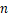个输入x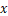=(x1,x2,…,xn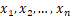)，对应n个权值w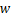=(w1,w2,…,wn)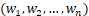，此外还有一个偏置项阈值，就是图中的b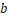，神经元将所有输入参数与对应权值进行加权求和，得到的结果经过激活函数变换后输出，计算公式如下：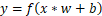2 讨论BP神经网络的学习过程
BP神经网络训练过程的基本步骤可以归纳如下：初始化网络权值和神经元的阈值，一般通过随机的方式进行初始化；前向传播:计算隐层神经元和输出层神经元的输出；后向传播:根据目标函数公式修正权值wij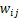。上述过程反复迭代，通过损失函数和成本函数对前向传播结果进行判定，并通过后向传播过程对权重参数进行修正，起到监督学习的作用，一直到满足终止条件为止。
3 BP神经网络有哪些常见应用?
BP网络主要用于以下四个方面。
函数逼近：用输入向量和相应的输出向量训练一个网络逼近一个函数。
模式识别：用一个待定的输出向量将它与输入向量联系起来。
分类：把输入向量所定义的合适方式进行分类。
数据压缩：减少输出向量维数以便于传输或存储
4 神经网络的激活函数有哪些？他们对神经网络的性能有何影响。
激活函数经常使用Sigmoid函数、tanh函数、ReLu 函数。
Sigmoid函数的优点在于输出范围有限，数据在传递的过程中不容易发散，并且其输出范围为(0,1)，可以在输出层表示概率值。Sigmoid函数的导数是非零的，很容易计算。
Sigmoid函数的主要缺点是梯度下降非常明显，且两头过于平坦，容易出现梯度消失的情况，输出的值域不对称，并非像tanh函数那样值域是-1到1。
双曲正切函数将数据映射到[-1,1]，解决了Sigmoid函数输出值域不对称问题。另外，它是完全可微分和反对称的，对称中心在原点。然而它的输出值域两头依旧过于平坦，梯度消失问题仍然存在。为了解决学习缓慢和梯度消失问题，可使用其更加平缓的变体，如log-log、Softsign、Symmetrical Sigmoid等
ReLU函数是目前神经网络里常用的激活函数，由于ReLU函数是线性特点使其收敛速度比Sigmoid、Tanh更快，而且没有梯度饱和的情况出现。计算更加高效，相比于Sigmoid、Tanh函数，只需要一个阈值就可以得到激活值，不需要对输入归一化来防止达到饱和。
5 BP神经网络训练过程中如何减少陷入最小极值点？
可以有多种方法：
以多组不同参数初始化多个神经网络，按标准方法训练后去其中误差最小的解作为最终参数。相当于从多个不同的初始点开始搜索，这样可能陷入不同的局部最小值，从中选出可能获得更接近全局最小的结果使用模拟退火。模拟退火在每一步都以一定概率接受比当前解更差的结果，从而有助于跳出局部最小。在每部迭代中接受次优解的概率随着时间的推移而逐渐降低，从而保证算法稳定采用随机梯度下降。与标准梯度下降的精确计算梯度不同，随机梯度下降在计算题都的时候加入了随机因素，因此，即时陷入局部极小点，它计算出来的梯度仍可能不为0，就有机会跳出局部最小继续搜索遗传算法

6 讨论BP神经网络的训练过程中学习不长、隐层个数、隐层单元数等参数如何调整？
算法的步长选择。步长实际上取值取决于数据样本，可以多取一些值，从大到小，分别运行算法，看看迭代效果，如果损失函数在变小，说明取值有效，否则要增大步长。步长太大，会导致迭代过快，甚至有可能错过最优解。步长太小，迭代速度太慢，很长时间算法都不能结束。所以算法的步长需要多次运行后才能得到一个较优的值。
网络的层数。理论已经证明，具有偏差和至少一个S型隐层加上一个线性输出层的网络，能够逼近任何有理函数，增加层数可以进一步降低误差，提高精度，但同时也是网络 复杂化。另外不能用仅具有非线性激活函数的单层网络来解决问题，因为能用单层网络解决的问题，用自适应线性网络也一定能解决，而且自适应线性网络的 运算速度更快，而对于只能用非线性函数解决的问题，单层精度又不够高，也只有增加层数才能达到期望的结果。
隐层单元数。在能够解决问题的前提下，再加上一两个神经元，以加快误差下降速度即可。
7 RBF神经网络的基本原理是什么?
径向基函数网络的隐含层是由径向基函数神经元组成，这一神经元的变换函数为径向基函数。典型的RBF网络由输入层、RBF隐层和由线性神经元组成的输出层。与传统的即神经网络相比，其主要区别是隐层节点中使用了径向基函数、对输入进行了高斯变换、将在原样本空间中的非线性问题，映射到高维空间中使其变得线性，然后在高维空间里用线性可分算法解决，RBF网络采用高斯函数作为核函数：
y=exp-bx-w2RBF网络的隐层神经元自带激活函数，所以其层数可以只有一层隐层， 权重值数量更少，所以RBF网络较BP网络速度快很多。
8 RBF为什么可以减少局部极少值难题？
当RBF的中心点确定以后，低维空间的输入通过非线性函数到一个高维空间的这种映射关系也就确定了。而隐含层空间到输出空间的映射是线性的，即网络的输出是隐单元输出的线性加权和，此处的权即为网络可调参数。由此可见，从总体上看，网络由输人到输出的映射是非线性的，而网络输出对可调参数而言却又是线性的。这样网络的权就可由线性方程组直接解出，从而大大加快学习速度并避免局部极小问题。
9 Elman神经网络的优点是什么?举例说明这种网络的应用
Elman网络是在时间上动态的，具有内部动态反馈的功能，承接层的设置使得Elman网络能够有效应对具有时变特征的数据，在带有时序性的样本数据上有着比静态神经网络更好的预测性能
10 与决策树比较，神经网络适合处理什么类型的数据和问题？
在中小数据集上，优先选择集成树模型。大数据集上推荐神经网络；在需要模型解释度的项目上，优先使用树模型；在项目时间较短的项目上，如果数据质量低(大量缺失值、噪音等)，优先使用集成树模型；在硬件条件有限及机器学习知识有限的前提下，优先选择树模型；对于结构化较高的数据，尤其是语音、图片、语言，优先使用神经网络模型（往往其数据量也较大）。
11 如何避免过拟合？
常用的防止过拟合的方法有参数范数惩罚、数据增强、提前终止、Bagging等集成方法、Dropout、批正则化等。
12 为什么要对模型的输入数据进行归一化？
归一化的目的就是使得预处理的数据被限定在一定的范围内（比如[0,1]或者[-1,1]），从而消除奇异样本数据导致的不良影响。
13 什么是梯度消失？
因为通常神经网络所用的激活函数是sigmoid函数，这个函数有个特点，就是能将负无穷到正无穷的数映射到0和1之间，并且对这个函数求导的结果是f′(x)=f(x)(1−f(x))。因此两个0到1之间的数相乘，得到的结果就会变得很小了。神经网络的反向传播是逐层对函数偏导相乘，因此当神经网络层数非常深的时候，最后一层产生的偏差就因为乘了很多的小于1的数而越来越小，最终就会变为0，从而导致层数比较浅的权重没有更新，这就是梯度消失。
14 如何加快梯度下降的速度？
可以使用mini-batch、Stochastic gradient descent等方法。mini-batch是将训练集分组，分组之后，分别对每组求梯度，然后更新参数。加入分 8组，则每次迭代将会做8次梯度下降，更新8次参数。所以mini-batch比传统的梯度下降法下降的速度快。Stochastic gradient descent可以看做是mini-batch的一种特殊情况，当mini-batch size等于1时，mini-batch就退化为Stochastic gradient descent。此时每次迭代中，对于数据集中每个样本都做一次梯度下降。还可以使用Monmentum、RMSprop、Adam等方法。
展开全文• 转自 ...Word2vec是一个将单词表征成向量的形式，它可以把文本内容的处理简化为向量空间中的向量运算，计算出向量空间上的相似度，来表示文本语义上的相似度。 Word2vec采用CBOW（Conti...
转自 https://blog.csdn.net/eastmount/article/details/49591349
最近在做知识图谱实体对齐和属性对齐中，简单用了下Word2vec谷歌开源代码。Word2vec是一个将单词表征成向量的形式，它可以把文本内容的处理简化为向量空间中的向量运算，计算出向量空间上的相似度，来表示文本语义上的相似度。
Word2vec采用CBOW（Continuous Bag-Of-Words Model，连续词袋模型）和Skip-Gram（Continuous Skip-GramModel）两种模型，涉及到神经网络和深度学习的一些知识。故这周给学弟和同学们分享PPT的主题就是《神经网络是个什么东东？》，参考了很多资料并简单讲述了机器学习和神经网络的入门知识。
希望文章对你有所帮助，文章中分享了很多参考资料和最后对吴老的赞扬~

一. 机器学习入门介绍
以前转载过"数盟"的一篇关于机器学习入门介绍的文章，这里简单引入它的几张图片作为引入。参考原文地址和转载地址：         机器学习科普文章：“一文读懂机器学习，大数据/自然语言处理/算法全有了”         http://blog.csdn.net/eastmount/article/details/43673209
机器学习这个词是让人疑惑的，首先它是英文名称Machine Learning（简称ML）的直译，在计算界Machine一般指计算机。这个名字使用了拟人的手法，说明了这门技术是让机器“学习”的技术。但是计算机是死的，怎么可能像人类一样“学习”呢？         下图是非常形象的将机器学习的过程与人类对历史经验归纳的过程做个比对：         机器学习方法是计算机利用已有的数据(经验)，得出了某种模型(迟到的规律)，并利用此模型预测未来(是否迟到)的一种方法。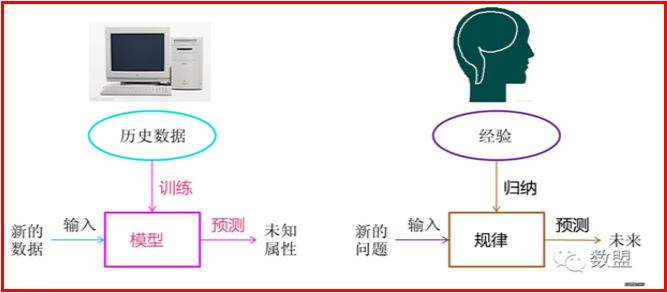常见的例子包括房价与面积、预测患病概率等等，详见斯坦福NG教授课程。        下图是机器学习所牵扯的一些相关范围的学科与研究领域。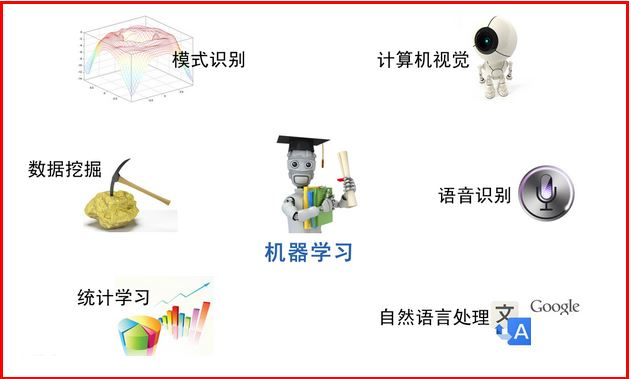简单的等价划分如下：        模式识别=机器学习: 两者的主要区别在于前者是从工业界发展起来的概念，后者则主要源自计算机学科。        数据挖掘=机器学习+数据库: 从数据中挖出金子，以及将废弃的数据转化为价值。        计算机视觉=图像处理+机器学习: 图像处理技术用于将图像处理为适合进入机器学习模型中的输入，机器学习则负责从图像中识别出相关的模式。例如百度识图、手写字符识别、车牌识别等等应用。        统计学习≈机器学习: 统计学习是个与机器学习高度重叠的学科。因为机器学习中的大多数方法来自统计学，甚至可认为统计学的发展促进机器学习的繁荣昌盛。例如著名的支持向量机算法，就是源自统计学科。区别在于统计学习者重点关注的是统计模型的发展与优化，偏数 学，而机器学习者更关注的是能够解决问题，偏实践。        语音识别=语音处理+机器学习: 语音识别就是音频处理技术与机器学习的结合。一般会结合自然语言处理的相关技术，目前的相关应用有苹果的语音助手siri。        自然语言处理=文本处理+机器学习:
让机器理解人类的语言，NLP中大量使用了编译原理相关的技术，例如词法分析、语法分析、语义理解等。
自然语言处理作为唯一由人类自身创造的符号，一直是机器学习界不断 研究的方向。按照百度机器学习专家余凯的说法“听与看，说白了就是阿猫和阿狗都会的，而只有语言才是人类独有的”。如何利用机器学习技术进行自然语言的的深度理解，一直是工业和学术界关注的焦点。
Deep Learning （深度学习）算法已经在图像和音频领域取得了惊人的成果，但是在 NLP 领域中尚未见到如此激动人心的结果。有一种说法是：
语言（词、句子、篇章等）属于人类认知过程中产生的高层认知抽象实体，而语音和图像属于较为底层的原始输入信号，所以后两者更适合做deep learning来学习特征。
而将词用“词向量”的方式表示可谓是将 Deep Learning 算法引入 NLP 领域的一个核心技术。大多数宣称用了 Deep Learning 的论文，其中往往也用了词向量。显然Word2vec也引入了词向量。参考licstar文章：

Deep Learning in NLP （一）词向量和语言模型

机器学习的方法很多，其中经典的方法包括：回归算法、神经网络、支持向量机SVM、聚类算法、降维算法、推荐算法、朴素贝叶斯、决策树等。详见这两篇文章：        常见的机器学习&数据挖掘知识点 - 作者：一只鸟的天空        [转载] 机器学习面试之算法思想简单梳理

二. 神经网络入门介绍
该部分主要通过白话文的方式讲述神经网络，其中主要转载吴老的文章。链接：        吴祖增前辈：神经网络入门(连载之一)        吴祖增前辈：神经网络入门(连载之二)        斯坦福机器学习视频NG教授 https://class.coursera.org/ml/class/index        书籍《游戏开发中的人工智能》、《游戏编程中的人工智能技术》        神经网络(也称之为人工神经网络，ANN)算法是80年代机器学习界非常流行的算法，不过在90年代中途衰落。现在，携着“深度学习”之势，神经网络重装归来，重新成为最强大的机器学习算法之一。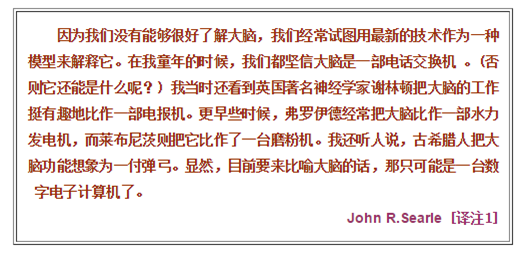人工神经网络（artificial neural network，缩写ANN），是一种模仿生物神经网络的结构和功能的数学模型或计算模型。神经网络由大量的人工神经元联结进行计算。其来源于生物，故吴老先先讲述了生物神经网络的基础知识，从而进行引入。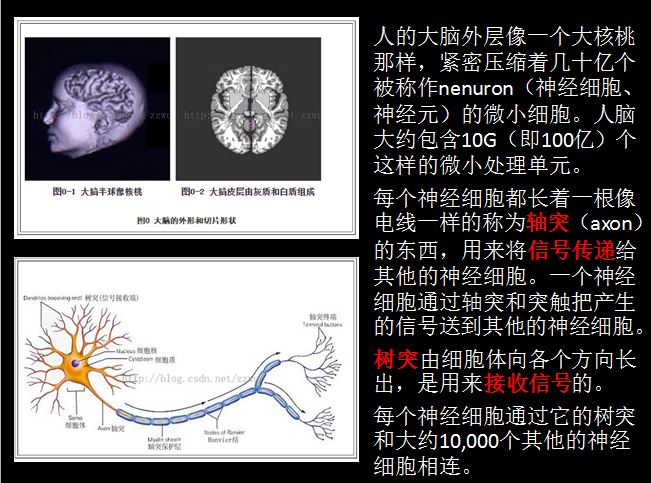神经细胞通过轴突将信号传递给其他的神经细胞，通过树突向各个方向接受信号。        神经细胞利用电-化学过程交换信号。输入信号来自另一些神经细胞。这些神经细胞的轴突末梢（也就是终端）和本神经细胞的树突相遇形成突触（synapse），信号就从树突上的突触进入本细胞。        信号在大脑中实际怎样传输是一个相当复杂的过程，但就我们而言，重要的是把它看成和现代的计算机一样，利用一系列的0和1来进行操作。就是说，大脑的神经细胞也只有两种状态：兴奋（fire）和不兴奋（即抑制）。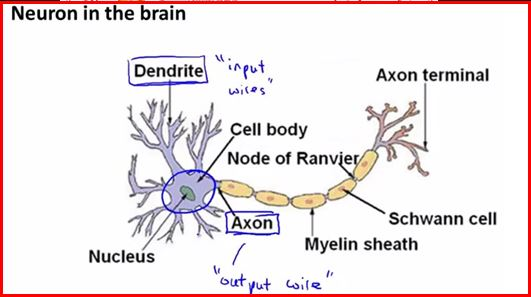神经细胞利用一种我们还不知道的方法，把所有从树突突触上进来的信号进行相加，如果全部信号的总和超过某个阀值，就会激发神经细胞进入兴奋（fire）状态，这时就会有一个电信号通过轴突发送出去给其他神经细胞。如果信号总和没有达到阀值，神经细胞就不会兴奋起来。这样的解释有点过分简单化，但已能满足我们的目的。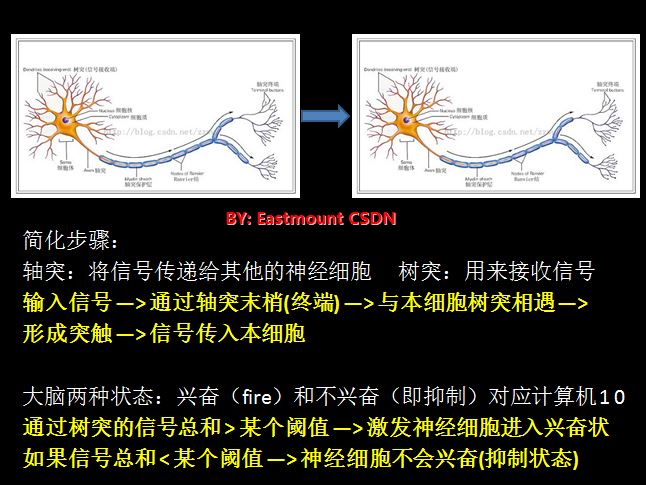由于人脑具有一下几个特点：        1.能实现无监督的学习        大脑能够自己进行学习，而不需要导师的监督教导。如果一个神经细胞在一段时间内受到高频率的刺激，则它和输入信号的神经细胞之间的连接强度就会按某种过程改变，使得该神经细胞下一次受到激励时更容易兴奋。        2.对损伤有冗余性(tolerance)        大脑即使有很大一部分受到了损伤, 它仍然能够执行复杂的工作。        3.处理信息的效率极高        神经细胞之间电-化学信号的传递，与一台数字计算机中CPU的数据传输相比，速度是非常慢的，但因神经细胞采用了并行的工作方式，使得大脑能够同时处理大量的数据。例如，大脑视觉皮层在处理通过我们的视网膜输入的一幅图象信号时，大约只要100ms的时间就能完成，眼睛并发执行。        4.善于归纳推广        大脑和数字计算机不同，它极擅长的事情之一就是模式识别，并能根据已熟悉信息进行归纳推广(generlize)。例如，我们能够阅读他人所写的手稿上的文字，即使我们以前从来没见过他所写的东西。

5.它是有意识的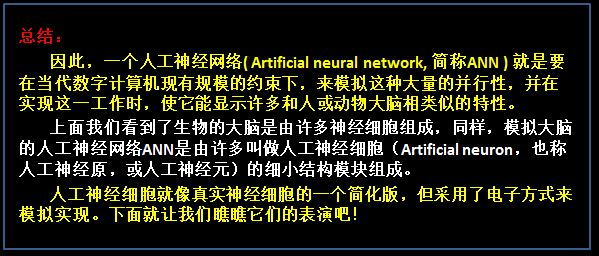如下图所示，它表示的是一个人工神经细胞。其中：        输入(Input)；权重(Weight)：左边五个灰色圆底字母w代表浮点数；激励函数(Activation Function)：大圆，所有经过权重调整后的输入加起来，形成单个的激励值；输出(Output)：神经细胞的输出。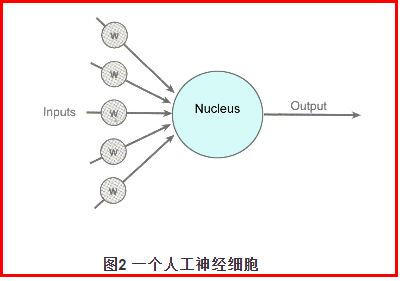进入人工神经细胞的每一个input(输入)都与一个权重w相联系，正是这些权重将决定神经网络的整体活跃性。假设权重为-1和1之间的一个随机数，权重可正可负（激发和抑制作用）。当输入信号进入神经细胞时，它们的值将与它们对应的权重相乘，作为图中大圆的输入。如果激励值超过某个阀值（假设阀值为1.0），就会产生一个值为1的信号输出；如果激励值小于阀值1.0，则输出一个0。这是人工神经细胞激励函数的一种最简单的类型。涉及的数学知识如下图所示：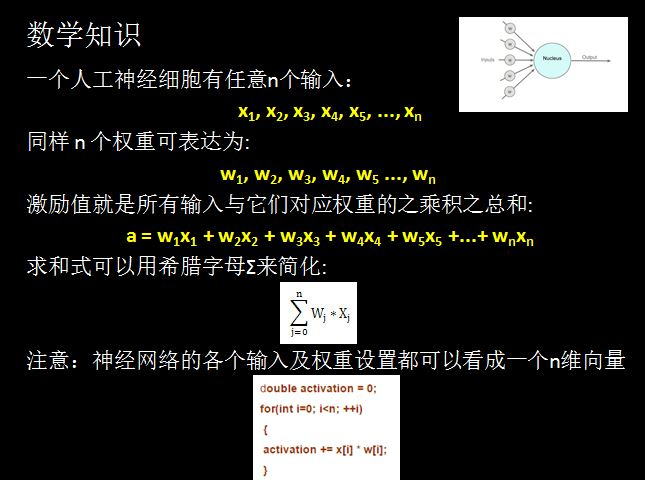如果最后计算的结果激励值大于阈值1.0，则神经细胞就输出1；如果激励值小于阈值则输出0。这和一个生物神经细胞的兴奋状态或抑制状态是等价的。下面图是通过神经网络实现逻辑表达式与运算：（参考NG斯坦福机器学习讲义）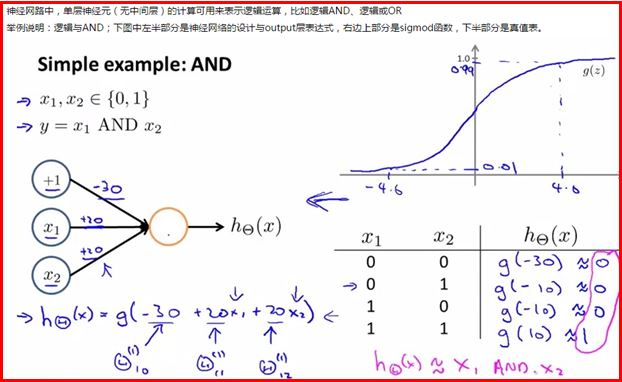可以看到x1和x2变量作为神经网络的输入，当它们取不同的0或1值时，其结果通过sigmod函数计算的值是不同的。它模拟了整个AND运算。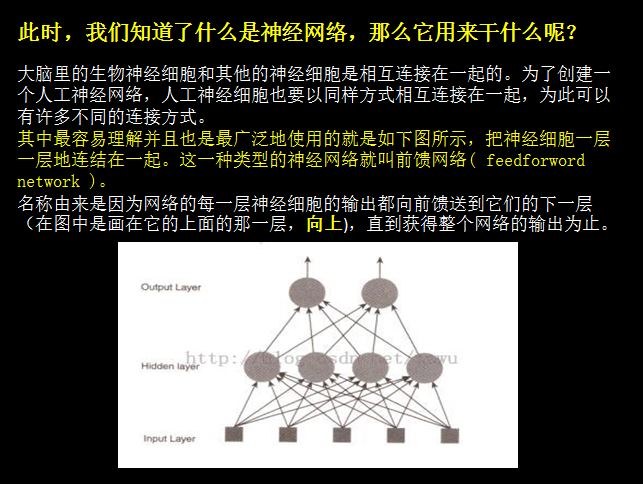该图中神经网络共有三层 ( 注输入层不是神经细胞，神经细胞只有两层 )：        输入层中的每个输入都馈送到了隐藏层，作为该层每一个神经细胞的输入；然后，从隐藏层的每个神经细胞的输出都连到了它下一层（即输出层）的每一个神经细胞。        注意：        1.图中仅仅画了一个隐藏层，作为前馈网络，一般地可以有任意多个隐藏层。但在对付你将处理的大多数问题时一层通常是足够的。        2.事实上，有一些问题甚至根本不需要任何隐藏单元，你只要把那些输入直接连结到输出神经细胞就行了。        3.每一层实际都可以有任何数目的神经细胞，这完全取决于要解决的问题的复杂性。但神经细胞数目愈多，网络的工作速度也就愈低，网络的规模总是要求保持尽可能的小。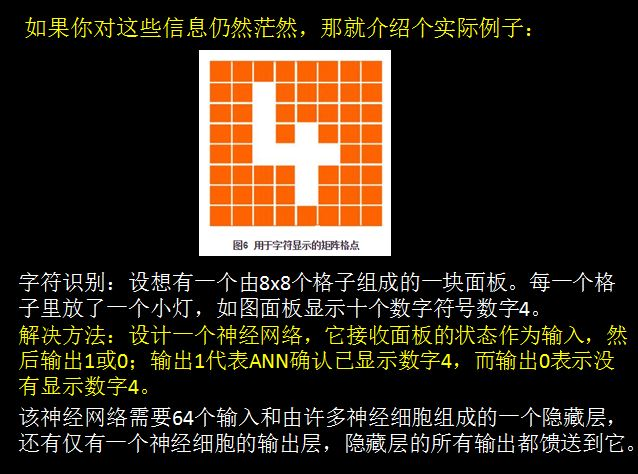神经网络体系创建成功后，它必须接受训练来认出数字4，方法：        1.先把神经网络的所有权重初始化为任意值；        2.然后给他一系列输入代表面板不同配置的输入，对每种输入配置，检查它的输出是什么，并调整相应的权重；        3.如果我们送给网络的输入模式不是4，则我们知道网络应该输出一个0。因此每个非4字符时，网络权重应进行调整，使得它的输出趋向于0；当代表4的模式输送给网络时，则应把权重调整到使其输出趋向于1；        4.我们可以进一步识别0到9的所有数字或字母，其本质是手写识别的工作原理。
5.最后，网络不单能认识已经训练的笔迹，还显示了它有显著的归纳和推广能力。

正是这种归纳推广能力，使得神经网络已经成为能够用于无数应用的一种无价的工具，从人脸识别、医学诊断，直到跑马赛的预测，另外还有电脑游戏中的bot（作为游戏角色的机器人）的导航，或者硬件的robot（真正的机器人）的导航。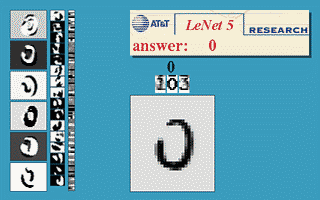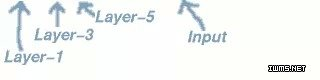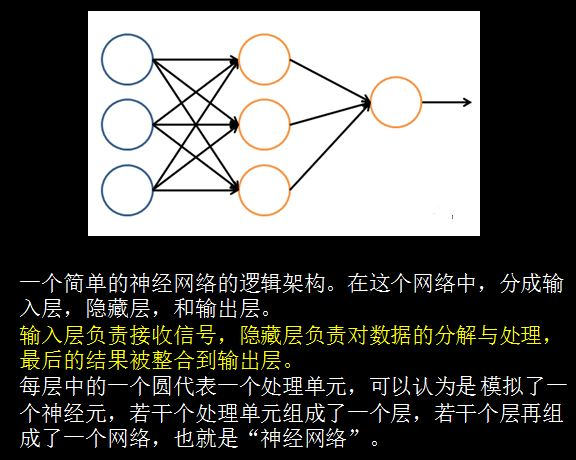最后给大家看一个利用神经网络对图片进行分类的例子：过程就不详细论述了，图片很清晰，对人、汽车、摩托车、卡车进行图片识别，而具体的隐藏层函数需要大家去深入研究，我自己研究得也很浅显，抱歉~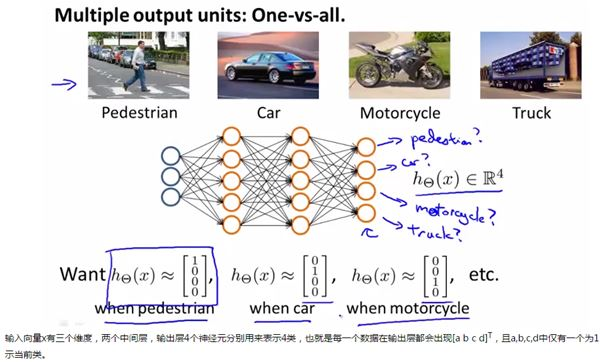参考资料包括NG教授的课程讲义和CSDN一位很厉害的女博主Rachel-Zhang：        Stanford机器学习---第五讲. 神经网络的学习 Neural Networks learning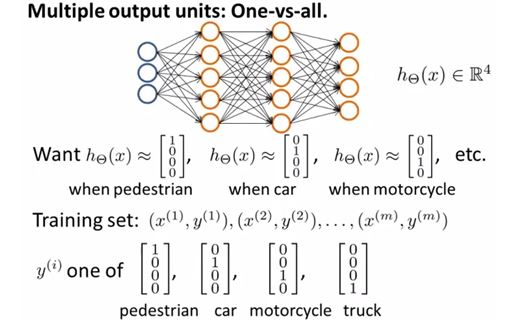三. 监督学习和无监督学习
因为给刚入学的学弟们讲的PPT，所以也简单讲述了监督学习和无监督学习的区别。下图是刘斌《Web数据挖掘》书的整体框架，当年我讲过。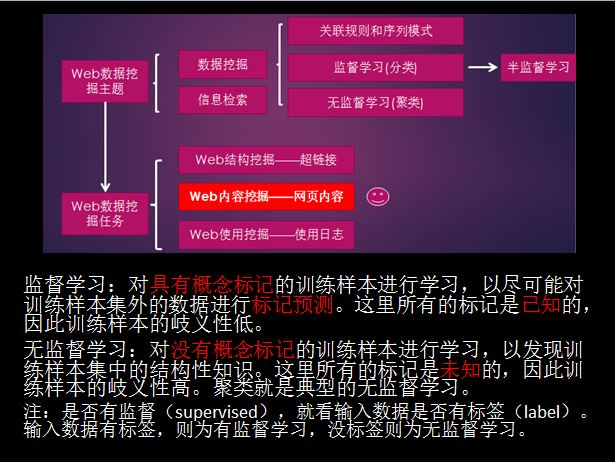在给它们解释监督学习和无监督学习的过程中，主要通过知乎的五个问题进行讲解。        第一个问题：什么是学习(Learning)？        一个成语就可以概括：举一反三 。以高考为例，高考的题目在上考场前我们未必做过，但在高三做过很多题目，懂得解题方法，因此考场上也能算出答案。        机器学习的思路类似：我们能不能利用一些训练数据(已经做过的题)使机器能够利用它们(解题方法)分析未知数据(高考题目)。

第二个问题：最普遍也是最简单的机器学习算法？

分类(Classification)：输入的训练数据有特征(feature)，有标签(label)。学习的本质就是找到特征和标签间的关系(mapping)。这样当有特征而无标签的未知数据输入时，我们可以通过已有的关系得到未知数据的标签。        第三个问题：上述分类所有训练数据都有标签，如果没有呢？         所有训练数据都有标签则为有监督学习(Supervised Learning)，如果数据没有标签则是无监督学习(Unsupervised Learning)，也即聚类(Clustering)。但有监督学习并非全是分类还有回归(Regression)。        无监督学习本身的特点使其难以得到如分类一样近乎完美的结果。这也正如我们在高中做题，答案(标签)是非常重要的，假设两个完全相同的人进入高中，一个正常学习，另一人做的所有题目都没有答案，那么想必第一个人高考会发挥更好，第二个人会发疯。        第四个问题：既然分类如此之好，聚类如此之不靠谱，那为何我们还可以容忍聚类的存在？        在实际应用中，标签的获取常常需要极大的人工工作量，有时甚至非常困难。例如在自然语言处理NLP中，Penn Chinese Treebank在2年里只完成了4000句话的标签。        第五个问题：难道有监督学习和无监督学习就是非黑即白的关系吗？有没有灰呢？         Good idea。灰是存在的。二者的中间带就是半监督学习(semi-supervised learning)。对于半监督学习，其训练数据的一部分是有标签的，另一部分没有标签，而没标签数据的数量常常极大于有标签数据数量(这也是符合现实情况的)。在你的人生路上，总有很多人说你这也不行，那也不行，梦想是你自己的；有梦想就需要学会自己去保护它。


展开全文• 计算出输出与标签间的损失函数值，然后计算其相对于每个神经元的梯度，根据梯度方向更新权值。 （1）将训练集数据输入到ANN的输入层，经过隐藏层，最后达到输出层并输出结果，这是ANN的前向传播过程； （2）...
• 6.2 以下是几种在神经网络或深度学习网络中常用的激活函数，试总结激活函数所具备的特征，并解释下面几种函数是否适合作为激活。 答： 激活函数一般特征： 非线性： 激活函数为非线性激活函数的时...
• 4.0.神经网络学习 1）题目： ...在本练习中，将自己实现反向传播算法来学习神经网络的参数。 本次的数据与上次作业是一样的，这里不再赘述。 数据集链接: https://pan.baidu.com/s/1i_i77cVsa5Tt...BP算法 吴恩达
• 网易云课堂上吴恩达教授的《神经网络与深度学习》的课程，第一周的测试习题
• 神经网络学习过程，就是根据训练数据来调整神经元之间的连接权（connection weight）以及每个功能神经元的阈值，神经网络经过学习后的东西，蕴藏在连接权与阈值中。 5.1神经元模型 其最初是模拟的生物神经元。...网络 算法 python
• 本文由 沈庆阳 所有,转载请与作者取得联系! 前言 卷积网络的应用并非始于近期，...卷积神经网络（CNN，Convolutional Neural Networks）是一种前馈神经网络，它的人工神经元可以响应一部分覆盖范围内的周围单元...人工智能 TensorFlow AI
• BAT机器学习面试1000系列 1 前言 1 BAT机器学习面试1000系列 2 1 归一化为什么能提高梯度下降法求解最优解的速度？ 22 2 归一化有可能提高精度 22 3 归一化的类型 23 1）线性归一化 23 2）标准差标准化 ...
• 3.1.前馈神经网络 1）题目： 在本次练习中，你将使用前馈神经网络来识别手写数字(从0到9)。数据依然是MNIST手写体数据集，这里包含了5000个训练样本。之前用了逻辑回归进行多元分类，这次用前馈神经网络进行预测。...吴恩达
• 作业下载 地址：... 1. sigmoid梯度计算 function g = sigmoidGradient(z) %SIGMOIDGRADIENT returns the gradient of the sigmoid function %evaluated at z % g...斯坦福大学 吴恩达
• 前言博主第一次接触机器学习，内容...正文周志华的机器学习第五章讲的是关于神经网络这一方面，内容上包括了基本神经网络的组成与基本概念，常见的神经网络学习算法以及相关优化问题，在后面简单介绍了几种常见的神...
• 请使用神经网络模型完成该数据集的分类。具体要求如下： 完成数据集的读取，洗牌，并将数据集合理分割成训练集和测试集 实现激活函数及其导数 实现代价函数 实现梯度下降并记录代价函数 完成模型的训练，并...多分类 手写数字识别
• Several exercises will make use of the following three-dimensional data sampled from three categories, denoted ωi
• 神经网络1.1各个激活函数的优缺点？1.2 为什么ReLU常用于神经网络的激活函数？1.3 梯度消失和梯度爆炸的解决方案？梯度爆炸引发的问题？1.4如何确定是否出现梯度爆炸？1.5神经网络中有哪些正则化技术？1.6 批量归一...计算机视觉 算法 tensorflow
• 我们在计算神经网络预测结果时采用了正向传播方法，从第一层开始正向一层一层进行计算算，直到最后一层的hθ(x)h_\theta (x)hθ​(x)。在不作正则化处理的情况下，逻辑回归中的代价函数如下所示： J(θ)=−1m[∑j=1...反向传播算法
• 机器学习计算力学的结合及应用初探 》论文学习 本文是对《机器学习计算力学的结合及应用初探 》论文的一个知识点的整理。 摘要： 1. 介绍了机器学习、数据科学与计算力学相结合的3种形式: 第一种是与有限...卷积RBF网络 异或问题 MATLAB代码
• 作者 |我是韩小琦链接 |https://zhuanlan.zhihu.com/p/476168485.1 试述将线性函数用作神经元激活函数的缺陷。答：使用线性函数作为激活函数时...
• 卷积神经网络同多层感知机（MLP）一样，通过设置多个隐层来实现对复杂模型的学习。如下图所示是一个手写字符识别的卷积神经网络结构示意图（书p114）： 从图中可以看到卷积层（convolutional layer）和采样层...theano mnist
• 4. RNNs和FNNs(前馈神经网络)有什么区别？5. RNNs训练和传统ANN训练异同点？6. 为什么RNN 训练的时候Loss波动很大7. 描述RNN的前向输出流程8. RNN中为什么会出现梯度消失,如何解决？9. LSTM核心思想图解10. LSTMs与...
• Coursera 吴恩达机器学习 课后作业 3/8python 逻辑回归 分类算法
• 现在很多人都说，做it门槛很低，脑子灵活点，愿意去熬的，培训个几个月就可以，无非是调调...(类)神经网络算法在深度学习等领域应用十分广泛。 而负反馈BP神经网络其中应用最多的一种，一起来试试手算理解神经网络吧。算法
• 高维情况依然可以用牛顿迭代求解, 但是问题是Hessian矩阵引入的复杂性, 使得牛顿迭代求解的难度大大增加, 但是已经有了解决这个问题的办法就是Quasi-Newton method, 不再直接计算hessian矩阵, 而是每一步的时候使用...深度学习
• 文章目录1.[神经网络](%28https://blog.csdn.net/cc13186851239/article/details/113983560%29)2.CNN2.1卷积神经网络的结构2.2 Keras搭建CNN2.2经典网络分类2.2.1 LeNet2.2.2 AlexNet2.2.2.1 AlexNet 对比LeNet 的...卷积 tensorflow...